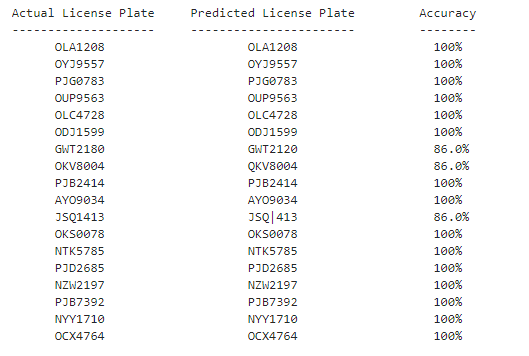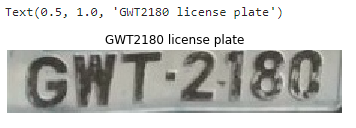Related Articles
License Plate Recognition with OpenCV and Tesseract OCR
• Difficulty Level : Basic
• Last Updated : 17 Jul, 2020

You will learn about Automatic number-plate recognition. We will use the Tesseract OCR An Optical Character Recognition Engine (OCR Engine) to automatically recognize text in vehicle registration plates.

Python-tesseract:
Py-tesseract is an optical character recognition (OCR) tool for python. That is, it’ll recognize and “read” the text embedded in images. Python-tesseract is a wrapper for Google’s Tesseract-OCR Engine. It is also used as an individual script, because it can read all image types like jpeg, png, gif, bmp, tiff, etc. Additionally, if used as a script, Python-tesseract will print the recognized text rather than writing it to a file. It has ability to recognize more than 100 languages.

Installation:

```pip install pytesseract
```

OpenCV:
OpenCV is an open source computer vision library. The library has more than 2500 optimized algorithms. These algorithms are often used to search and recognize faces, identify objects, recognize scenery and generate markers to overlay images using augmented reality, etc.

Installation:

```pip install opencv-python
```

Note: make sure you installed pytesseract and OpenCV-python modules properly
Note: you should have the dataset ready and all images should be as shown below in image processing techniques for best performance; dataset folder should be in same folder as you are writing this python code in or you will have to specify the path to dataset manually wherever needed.

Procedure:

 `# Loading the required python modules ` `import` `pytesseract ``# this is tesseract module ` `import` `matplotlib.pyplot as plt ` `import` `cv2 ``# this is opencv module ` `import` `glob ` `import` `os`

Note: the name of image files has to be the exact number in respective license plate image. example: if you have a with license plate having number as “FTY349U” then name the image file as “FTY349U.jpg”.

Code: Perform OCR using the Tesseract Engine on license plates

 `# specify path to the license plate images folder as shown below ` `path_for_license_plates ``=` `os.getcwd() ``+` `"/license-plates/**/*.jpg"` `list_license_plates ``=` `[] ` `predicted_license_plates ``=` `[] ` ` `  `for` `path_to_license_plate ``in` `glob.glob(path_for_license_plates, recursive ``=` `True``): ` `     `  `    ``license_plate_file ``=` `path_to_license_plate.split(``"/"``)[``-``1``] ` `    ``license_plate, _ ``=` `os.path.splitext(license_plate_file) ` `    ``''' ` `    ``Here we append the actual license plate to a list ` `    ``'''` `    ``list_license_plates.append(license_plate) ` `     `  `    ``''' ` `    ``Read each license plate image file using openCV ` `    ``'''` `    ``img ``=` `cv2.imread(path_to_license_plate) ` `     `  `    ``''' ` `    ``We then pass each license plate image file ` `    ``to the Tesseract OCR engine using the Python library  ` `    ``wrapper for it. We get back predicted_result for  ` `    ``license plate. We append the predicted_result in a ` `    ``list and compare it with the original the license plate ` `    ``'''` `    ``predicted_result ``=` `pytesseract.image_to_string(img, lang ``=``'eng'``, ` `    ``config ``=``'--oem 3 --psm 6 -c tessedit_char_whitelist = ABCDEFGHIJKLMNOPQRSTUVWXYZ0123456789'``) ` `     `  `    ``filter_predicted_result ``=` `"``".join(predicted_result.split()).replace("``:``", "``").replace("``-``", "``") ` `    ``predicted_license_plates.append(filter_predicted_result) `

Now we have the plates predicted but we haven’t seen what is the prediction, so to view the data and prediction we do a bit of visualization as shown below. we are also calculating the accuracy of prediction without using any built-in function.

 `print``(``"Actual License Plate"``, ``"\t"``, ``"Predicted License Plate"``, ``"\t"``, ``"Accuracy"``) ` `print``(``"--------------------"``, ``"\t"``, ``"-----------------------"``, ``"\t"``, ``"--------"``) ` ` `  `def` `calculate_predicted_accuracy(actual_list, predicted_list): ` `    ``for` `actual_plate, predict_plate ``in` `zip``(actual_list, predicted_list): ` `        ``accuracy ``=` `"0 %"` `        ``num_matches ``=` `0` `        ``if` `actual_plate ``=``=` `predict_plate: ` `            ``accuracy ``=` `"100 %"` `        ``else``: ` `            ``if` `len``(actual_plate) ``=``=` `len``(predict_plate): ` `                ``for` `a, p ``in` `zip``(actual_plate, predict_plate): ` `                    ``if` `a ``=``=` `p: ` `                        ``num_matches ``+``=` `1` `                ``accuracy ``=` `str``(``round``((num_matches ``/` `len``(actual_plate)), ``2``) ``*` `100``) ` `                ``accuracy ``+``=` `"%"` `        ``print``(``"     "``, actual_plate, ``"\t\t\t"``, predict_plate, ``"\t\t  "``, accuracy) ` ` `  `         `  `calculate_predicted_accuracy(list_license_plates, predicted_license_plates)`

Output:We see that the Tesseract OCR engine mostly predicts all of the license plates correctly with 100% accuracy. For the license plates, the Tesseract OCR Engine predicted incorrectly (i.e. GWT2180, OKV8004, JSQ1413), we will apply image processing techniques on those license plate files and pass them to the Tesseract OCR again. Applying the image processing techniques would increase the accuracy of the Tesseract Engine for the license plates of GWT2180, OKV8004, JSQ1413.

Code: Image Processing Techniques

 `# Read the license plate file and display it ` `test_license_plate ``=` `cv2.imread(os.getcwd() ``+` `"/license-plates / GWT2180.jpg"``) ` `plt.imshow(test_license_plate) ` `plt.axis(``'off'``) ` `plt.title(``'GWT2180 license plate'``)`

Output:• Image resizing:

Resize the image file by a factor of 2x in both the horizontal and vertical directions using cv2.resize

 `resize_test_license_plate ``=` `cv2.resize( ` `    ``test_license_plate, ``None``, fx ``=` `2``, fy ``=` `2``,  ` `    ``interpolation ``=` `cv2.INTER_CUBIC)`

• Converting to Gray-scale: Next, we convert our resized image file to gray scale to optimize the detection and reduce the amount of colors present in image drastically which will help in the detection of license plates easily.

 `grayscale_resize_test_license_plate ``=` `cv2.cvtColor( ` `    ``resize_test_license_plate, cv2.COLOR_BGR2GRAY) `

• Denoising the Image:
Gaussian Blur is a technique for denoising images. it makes the edges more clearer and smoother which in-turn makes the characters more readable.

 `gaussian_blur_license_plate ``=` `cv2.GaussianBlur( ` `    ``grayscale_resize_test_license_plate, (``5``, ``5``), ``0``) `

Now, pass the transformed license plate file to the Tesseract OCR engine and see the predicted result.

 `new_predicted_result_GWT2180 ``=` `pytesseract.image_to_string(gaussian_blur_license_plate, lang ``=``'eng'``, ` `config ``=``'--oem 3 -l eng --psm 6 -c tessedit_char_whitelist = ABCDEFGHIJKLMNOPQRSTUVWXYZ0123456789'``) ` `filter_new_predicted_result_GWT2180 ``=` `"``".join(new_predicted_result_GWT2180.split()).replace("``:``", "``").replace("``-``", "``") ` `print``(filter_new_predicted_result_GWT2180)`

Output:

`GWT2180 `

Similarly, do this image processing for all other number plates that didn’t get 100% accuracy. Finally, the license plate detection model is ready.My Personal Notes arrow_drop_up
Recommended Articles
Page :